# MCAT Physical : Le Chatelier's Principle and Common Ion Effect

## Example Questions

← Previous 1 3

### Example Question #1 : Le Chatelier's Principle And Common Ion Effect

If the reactants and/or products in a chemical reaction are gases, the reaction rate can be determined by measuring the change of pressure as the reaction proceeds. Consider the following reaction and pressure vs. reaction rate data below.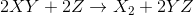Trial PXY(torr) PZ(torr) Rate (torr/s) 1 100 200 0.16 2 200 200 0.32 3 200 100 0.04 4 200 150 0.14

If the volume of the container were reduced, what would happen to the rate of the reaction?

Stay the same

Decrease

It depends on the pressure

Increase

Increase

Explanation:

Reducing the volume of the container increases pressure. This results in a higher frequency of gas particle collisions, thereby increasing the rate of the reaction.

### Example Question #2 : Le Chatelier's Principle And Common Ion Effect

Consider the following equation for the production of ammonia gas from hydrogen gas and nitrogen gas.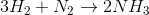If the volume of the vessel containing hydrogen and nitrogen is decreased, the production of ammonia __________.

is disfavored

is favored

is not affected

depends on original volume

is favored

Explanation:

Since decreasing the volume of the container has the effect of increasing pressure, equilibrium is shifted to the right. An increase in pressure has the result of favoring the side of the reaction with fewer moles of gas. (According to the balanced equation, there are 4 moles on the reactant side as opposed to 2 moles on the product side).

### Example Question #3 : Le Chatelier's Principle And Common Ion Effect

Barium fluoride dissolves in solution according to the following equation.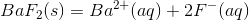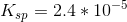Enough BaF2 is added to create a saturated liter of aqueous solution.

Suppose that 1M NaF is added to the solution, such that it does not change the volume of the solution. What would you expect  to change as a result of the addition of NaF?

The solubility of BaFwill decrease.

More BaF2 will dissolve.

The pH of the solution will decrease.

The solubility product constant will decrease.

The solubility of BaFwill decrease.

Explanation:

By adding NaF to the equation, 1M of F- ions are added to the solution. Thinking in terms of Le Chatlier's principle, the addition of a compound on one side of the equation will cause a shift to the other side of the reaction.

It DOES NOT affect the solubility product constant. Since there is an addition to the products side of the reaction, there will be a shift to the left, and more salt (reactant) will be precipitated. As a result, the solubility of the salt has decreased, because of the addition of NaF. This is known as the common ion effect.

### Example Question #1 : Le Chatelier's Principle And Common Ion Effect

The Haber-Bosch process, or simply the Haber process, is a common industrial reaction that generates ammonia from nitrogen and hydrogen gas. A worker in a company generates ammonia from the Haber process. He then dissociates the gaseous ammonia in water to produce an aqueous solution. Since ammonia is a base, it will accept a proton from water, generating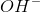and ammonium ion products. The two reactions involved are: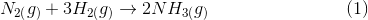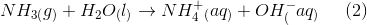The worker could do which of the following to shift the equilibrium towards hydroxide () ions?

II. Add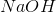III. Add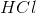I and II

I only

III only

I and III

I and III

Explanation:

You need to understand Le Chatelier’s principle to solve this question. Le Chatelier’s principle states that the addition of excess molecules to one side of a reaction will shift the equilibrium toward the other side. This shift makes sure that the reaction remains at equilibrium.

If you add hydrogen gas, reaction 1 will shift to the right and will produce more ammonia. Since more ammonia is produced, reaction 2 will shift to the right to produce more ammonium ions and hydroxide ions. Statement I is correct.

Addingto the solution will increase the concentration of hydroxide ions. This means that the equilibrium of reaction 2 will shift to the left, away from hydroxide ions. While the overall hydroxide ion concentration may increase due to the addition of the base, the equilibrium for the reaction will shift away from the hydroxide ions, making statement II incorrect.

Finally, adding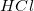will generate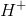ions. Theseions will react with theions and form water, which will decrease the overall concentration of hydroxide ions. The equilibrium of reaction 2 will shift to the right to produce more ammonium and hydroxide ions. Statement III is correct.

### Example Question #5 : Le Chatelier's Principle And Common Ion Effect

The Haber-Bosch process, or simply the Haber process, is a common industrial reaction that generates ammonia from nitrogen and hydrogen gas. A worker in a company generates ammonia from the Haber process. He then dissociates the gaseous ammonia in water to produce an aqueous solution. Since ammonia is a base, it will accept a proton from water, generatingand ammonium ion products. The two reactions involved are:What will happen if the worker increases the pH?

The equilibrium of reaction 2 will shift to the left

The amount of ammonium ions will increase

Changing the pH will have no effect

The amount of ammonia will stay constant

The equilibrium of reaction 2 will shift to the left

Explanation:

Increasing the pH is equivalent to decreasing hydrogen ion concentration or increasing hydroxide ion concentration. This increase in hydroxide ion concentration will shift the equilibrium of reaction 2 to the left, producing more ammonia. This is a fundamental point of Le Chatelier's principle.

### Example Question #6 : Le Chatelier's Principle And Common Ion Effect

Two solutions of the same ionic compound are prepared. One solution uses a pure water solvent, while the other uses a solution of dilute sodium chloride. Equal amounts of the ionic compound are added to each solvent. A student observes that a precipitate forms in the solution that used brine as a solvent, but not in the aqueous solution.

What is the most likely cause of this phenomenon?

Common-ion effect

A dissociation reaction occurred

Le Chatelier’s principle

The solution is saturated

Common-ion effect

Explanation:

This phenomenon is called the common-ion effect. When a compound dissolves in water it dissociates into ions. Increasing the concentration of one of these ions will shift the equilibrium towards the compound, thereby making it hard for the compound to dissolve in water (decreases solubility of compound). The ionic compound may have been something like silver chloride, which has a low solubility in water. A small amount may dissolve in aqueous solution, but the preexistence of chloride ions in the solvent would reduce the already low solubility and generate a precipitate.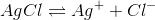Increasing chloride ion concentration shifts equilibrium to the left. While this phenomenon is directly linked to Le Chatelier's principle, the direct term is the common-ion effect.

A dissociation reaction does occur, but that does not explain the formation of the precipitate in only one solution. Also, since equal amounts of the ionic compounds are added to each solvent, we cannot make any conclusions about saturation.

### Example Question #7 : Le Chatelier's Principle And Common Ion Effect

Calcium carbonate dissolves in water based on the following reaction: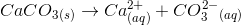Which of the following will decrease the solubility of the salt?

Add calcium chloride to the solution

Add more water to the solution

Remove calcium ions from the solution

Add calcium chloride to the solution

Explanation:

When thinking about the solubility of a salt, it helps to use Le Chatelier's principle. The solubility of a salt is dependent on the amount of ions that are created by the precipitate in solution. As a result, we decrease the solubility by increasing the amount of ions from the salt in solution.In this case, increasing the amount of calcium or carbonate ions will shift the reaction to the left, decreasing solubility.

The common-ion effect tells us that when an ion made by the salt is increased by another substance, the solubility of the salt will decrease. Calcium chloride will dissolve completely in solution, and will increase the amount of calcium ions. This will shift the reaction to the left, thus reducing the solubility of the precipitate.

### Example Question #8 : Le Chatelier's Principle And Common Ion Effect

Consider the reaction reaction below.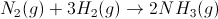A student allows the system to reach equilibrium and then removes two moles of hydrogen gas. Which of the following will be a result?

The reaction will shift to the side with fewer total moles of gas

More NH3 will be produced

No change will occur

The amount of N2 in the reaction vessel will increase

The reaction will first shift toward the products, then toward the reactants

The amount of N2 in the reaction vessel will increase

Explanation:

According to Le Chatelier's principle, when a system at equilibrium is disturbed, the system will react to restore equilibrium. In other words, it will seek to undo the stress. Here, if hydrogen gas is removed, the reaction will shift toward the reactants to re-form it. In the process, more nitrogen will be produced.

### Example Question #9 : Le Chatelier's Principle And Common Ion Effect

Carbonic anhydrase is an important enzyme that allows CO2 and H2O to be converted into H2CO3. In addition to allowing CO2 to be dissolved into the blood and transported to the lungs for exhalation, the products of the carbonic anhydrase reaction, H2CO3 and a related compound HCO3-, also serve to control the pH of the blood to prevent acidosis or alkalosis. The carbonic anhydrase reaction and acid-base reaction are presented below.

CO2 + H2O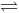H2CO3

H2CO3HCO3- + H+

The addition of H2CO3 would __________ the concentration of HCO3-.

not affect

decrease

increase

increase

Explanation:

This is a Le Chatlier shift problem. When the equilibrium of a chemical reaction is disturbed, the reaction shifts to the side to minimize the change. Here, increasing H2CO3 would shift the reaction to the right to minimize the addition of the acid. Shifting the reaction to the right would thus increase the concentration of HCO3-.

### Example Question #10 : Le Chatelier's Principle And Common Ion Effect

Carbonic anhydrase is an important enzyme that allows CO2 and H2O to be converted into H2CO3. In addition to allowing CO2 to be dissolved into the blood and transported to the lungs for exhalation, the products of the carbonic anhydrase reaction, H2CO3 and a related compound HCO3-, also serve to control the pH of the blood to prevent acidosis or alkalosis. The carbonic anhydrase reaction and acid-base reaction are presented below.

CO2 + H2OH2CO3

H2CO3HCO3- + H+

How would the addition of pure H2O shift the carbonic anhydrase reaction?

Rightward shift

No change

Leftward shift